# Quantitative Methods Overview DR SATISH NARGUNDKAR GEORGIA STATE

• Slides: 11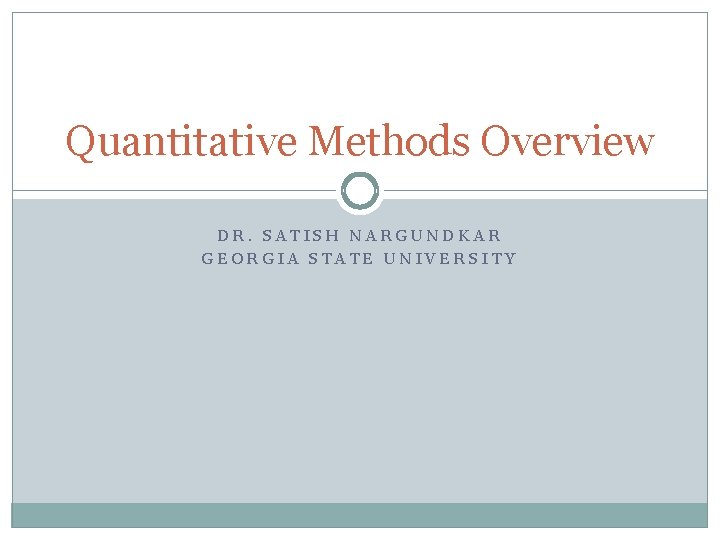Quantitative Methods Overview DR. SATISH NARGUNDKAR GEORGIA STATE UNIVERSITYReasoning �Deductive Theory testing General to specific True premise + sound logic = true conclusion �Inductive Theory building Specific to general True premise + sound logic = probability of a true conclusion �Abductive Best (most plausible) conclusion with available information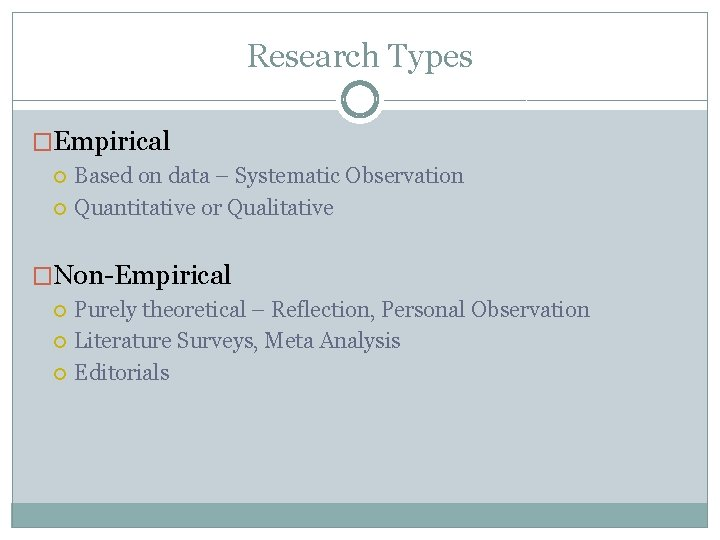Research Types �Empirical Based on data – Systematic Observation Quantitative or Qualitative �Non-Empirical Purely theoretical – Reflection, Personal Observation Literature Surveys, Meta Analysis Editorials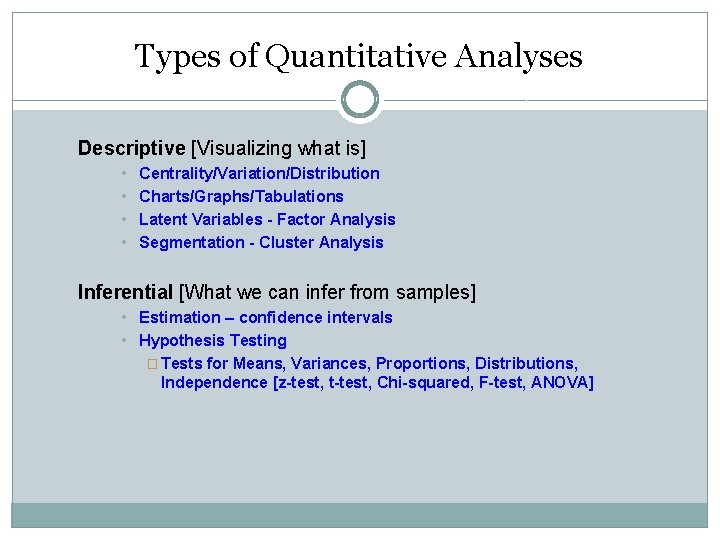Types of Quantitative Analyses Descriptive [Visualizing what is] • • Centrality/Variation/Distribution Charts/Graphs/Tabulations Latent Variables - Factor Analysis Segmentation - Cluster Analysis Inferential [What we can infer from samples] • Estimation – confidence intervals • Hypothesis Testing � Tests for Means, Variances, Proportions, Distributions, Independence [z-test, t-test, Chi-squared, F-test, ANOVA]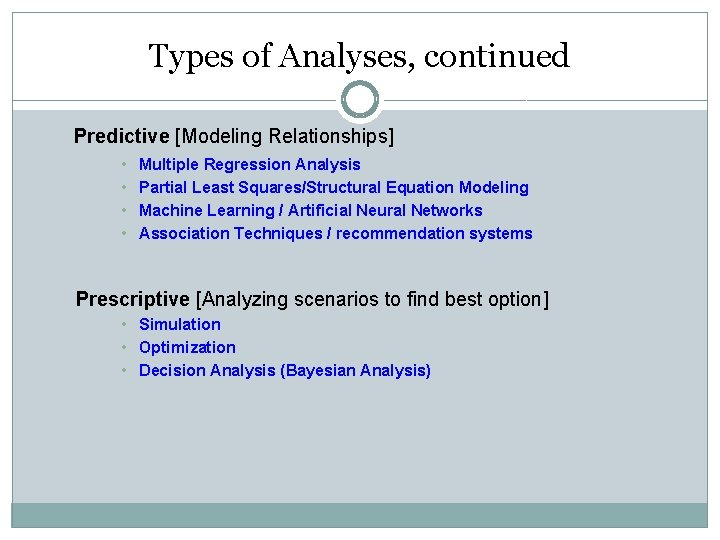Types of Analyses, continued Predictive [Modeling Relationships] • • Multiple Regression Analysis Partial Least Squares/Structural Equation Modeling Machine Learning / Artificial Neural Networks Association Techniques / recommendation systems Prescriptive [Analyzing scenarios to find best option] • Simulation • Optimization • Decision Analysis (Bayesian Analysis)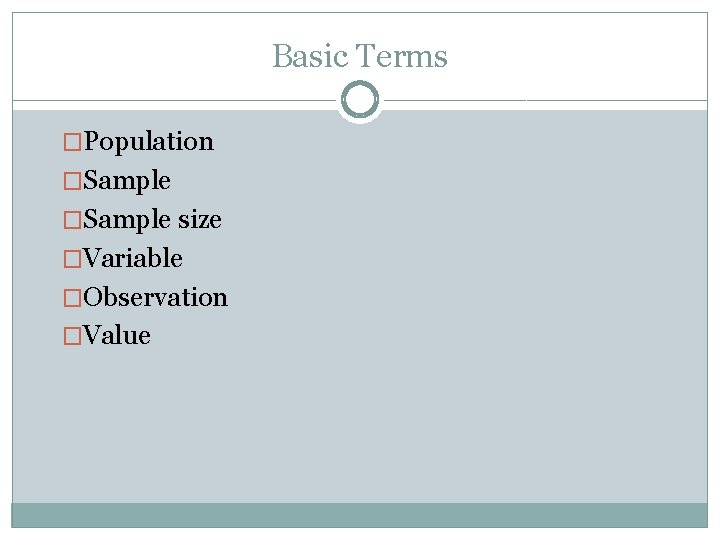Basic Terms �Population �Sample size �Variable �Observation �ValueData Scales �Nominal �Ordinal �Interval �Ratio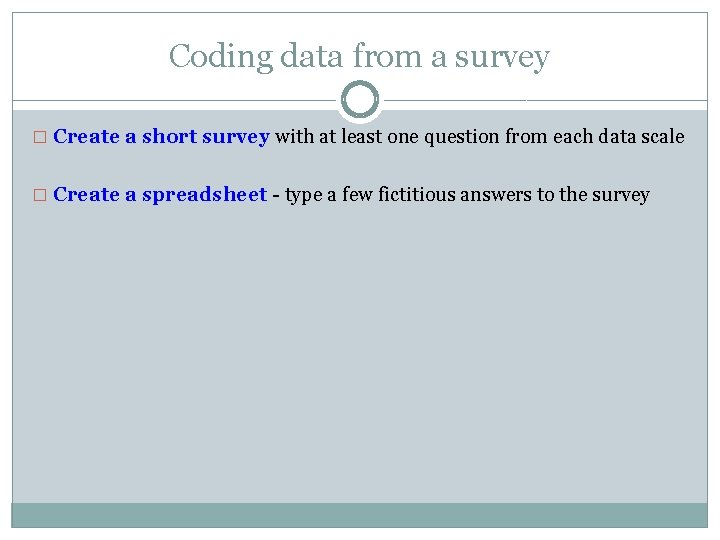Coding data from a survey � Create a short survey with at least one question from each data scale � Create a spreadsheet - type a few fictitious answers to the surveySequence of Analysis � Univariate – one variable at a time. Why? � Bivariate – dependent vs each independent. Why? � Multivariate – dependent vs all independents together.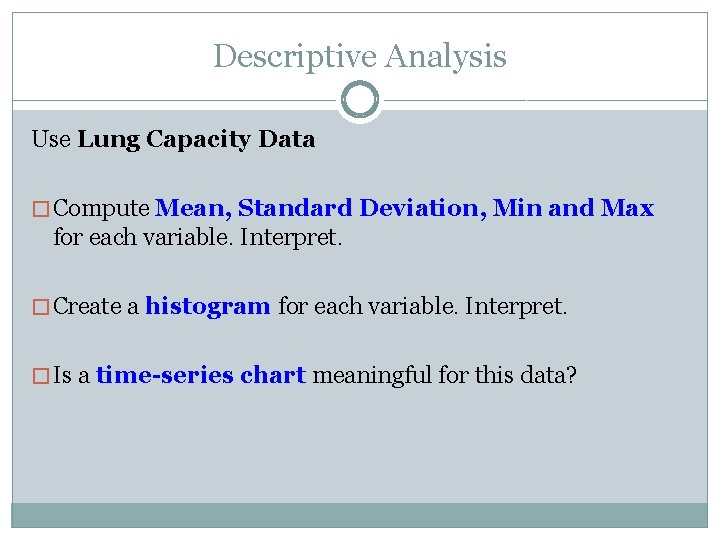Descriptive Analysis Use Lung Capacity Data � Compute Mean, Standard Deviation, Min and Max for each variable. Interpret. � Create a histogram for each variable. Interpret. � Is a time-series chart meaningful for this data?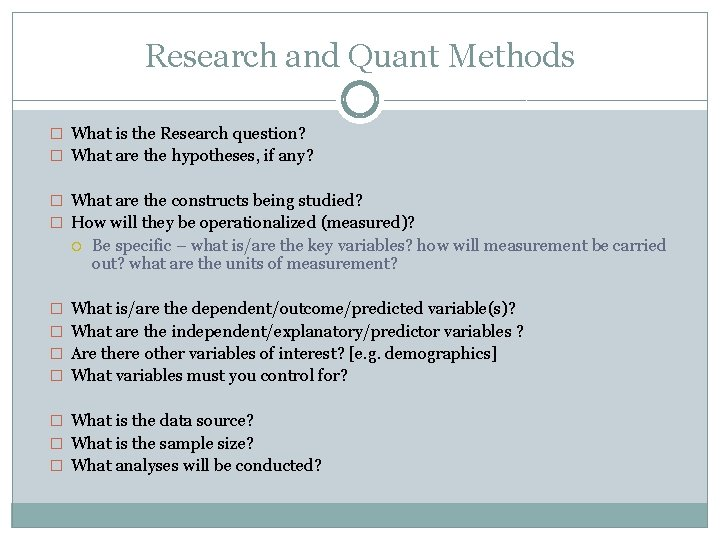Research and Quant Methods � What is the Research question? � What are the hypotheses, if any? � What are the constructs being studied? � How will they be operationalized (measured)? Be specific – what is/are the key variables? how will measurement be carried out? what are the units of measurement? � What is/are the dependent/outcome/predicted variable(s)? � What are the independent/explanatory/predictor variables ? � Are there other variables of interest? [e. g. demographics] � What variables must you control for? � What is the data source? � What is the sample size? � What analyses will be conducted?